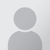CATEGORY
B4, 309-10, Spaze iTech Park, Sector 49, Sohna Road, 122001 Gurugram India

# The total amunt charged to my seller is 78.8 and tax applied on it is 18%, how can the charge on it become Rs. 20.8?Udit
StoreHippo expert
We have checked it at our end the tax applied on the total transaction was 36%, not 18% as the total tax applied is 18%+9%+9%  in your GST. As a resolution, you have to create new taxes of the name CGST, SGST, and IGST. And apply that given tax in the given format.

And as we calculated the tax on the product on which the total amount was 78.5.
As we know the taxes are included. So:
78.5= Actual amount + Taxed amount.
78.5= x + .36*x( where x is the the actual amount and tax is 36%)
78.5= 1.36*x
57.7= x
The total tax which was shown is Rs. 20.8.

Hence, Total amount= 57.7+20.8
= Rs. 78.5

Please create another tax which has 18% and apply it to the seller ledger rule as a workaround so that 36% tax does not get applied.
The issue is coming because the location of the admin is not being detected as of now. So, the system is not able to check whether to apply IGST or SGST+CGST to the respective rule. We have asked the team to work on this functionality and check the address based on the billing address. We will let you know once this functionality is implemented.

36% tax is applied because rule applied on your GST is 18%+9%+9%.

Sep 14, 2017 8:12:12 PM